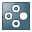Show TOCCost Function

TRUCK001 with means of transport TRUCK has a maximum loading capacity of 2500 cubic meters. Your company has the following business requirement: each truck is to leave the plant as full as possible. If this is not possible, the load must be at least 2000 cubic meters (minimum load). The following figure shows how you can use a cost function to map this business requirement:

You define two segments for this cost function:

• For the first segment, you define penalty costs of 1500 and a load of 0 cubic meters. You have also defined a gradient of 0 (zero). This means that if TRUCK001 leaves the plant with a load of less than 2000 cubic meters, the optimizer calculates penalty costs of 1500.

• For the second segment, you define penalty costs of 500 and a load of 2000 cubic meters. You also define a gradient of 1.000-. This means that if TRUCK001 leaves the plant with a load of between 2000 and 2500 cubic meters, the optimizer calculates penalty costs between 500 and 0 (zero) in accordance with the gradient (decreasing in intervals of one unit for each additional cubic meter loaded onto the truck).